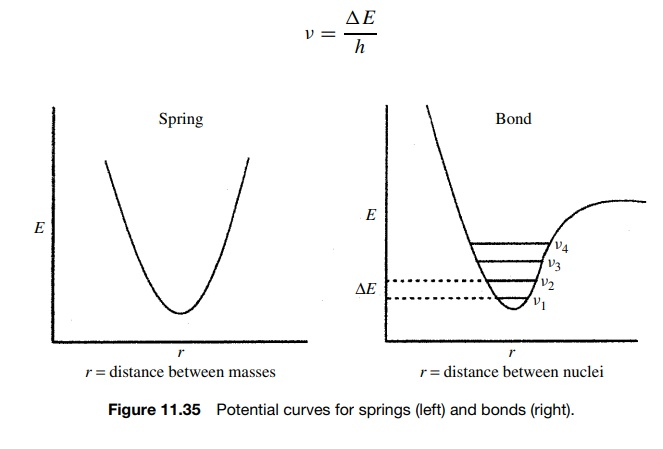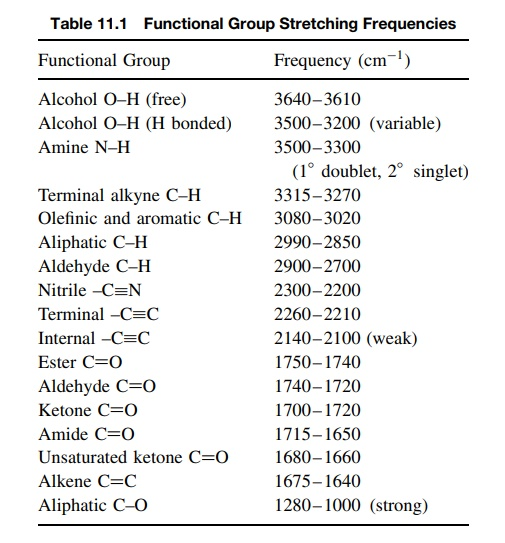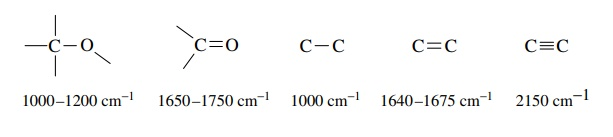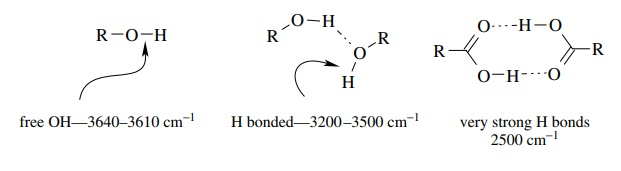# IR Stretching Frequencies

| Home | | Organic Chemistry |

## Chapter: Organic Chemistry : Structure Determination of Organic Compounds

As mentioned above, stretching modes are those in which internuclear distances change. For such a distance change to occur, the bond between the nuclei must be stretched or compressed.

IR STRETCHING FREQUENCIES

As mentioned above, stretching modes are those in which internuclear distances change. For such a distance change to occur, the bond between the nuclei must be stretched or compressed. One way to think about this is to consider a chemical bond as a spring connecting two masses (Figure 11.34). Each spring has a particular force constant which corresponds to the force required to compress or stretch that spring. Furthermore the frequency of vibration is depen-dent on the masses that the spring connects. For a given spring, heavy masses lead to lower frequency vibrations while light masses lead to higher frequency vibration.This analogy is actually very close to correct in describing stretching vibrations of bonds because the potential curves for springs and bonds have the same general shape and behavior at lower energies. The major difference is that the vibrational energies of a bond are quantized while those of a spring are not. As a result there are discrete vibrational energy levels that are allowed for a bond in a molecule. At room temperature, the vast majority of molecules are in the lowest vibrational energy levels.

To go from the lowest vibrational level ν1 to the next higher vibrational level ν2 (which is the only type of vibrational transition that is allowed), energy must be absorbed by the molecule which corresponds exactly to , the energy gap between the two vibrational levels (Figure 11.35). It follows that if the energy required to cause a vibrational transition is ΔE, then only light of the particular frequency and hence energy which corresponds to the energy of the vibrational transition will be absorbed. Moreover the frequency of light which causes the transition is given by

v = ΔE/hFor most bonds, these frequencies occur in the IR region. Each stretching mode in a molecule has its own potential curve and associated energy levels. Thus each stretching mode in a molecule will absorb IR energy at the particular frequency required to cause the transition from the lowest energy level to the next higher energy level. Since each of these energy levels is dependent upon the force constants of the bonds and the masses of the atoms they connect, each type of bond has a characteristic IR absorption corresponding to the stretching frequency of that bond.

Because functional groups are recurring groups of atoms connected by sim-ilar bonding patterns, a given functional group tends to give characteristic IR absorptions due to the vibrational frequencies of bonds present in that functional group. Infrared spectroscopy thus provides a fast and effective way to identify functional groups present in a molecule by noting the presence of absorptions corresponding to the bond types present in those functional groups. Table 11.1 is a compilation of IR absorptions for commonly encountered bonds and func-tional groups. Normal ranges are given since the absorption frequency of a given bond type can vary somewhat depending on the structure. The frequencies given are all stretching frequencies; bending frequencies are much more numerous and usually harder to interpret and are not included in this work.It is also possible to identify structural features in molecules which strengthen or weaken bonds and thus lead to shifts in IR frequencies. Several general effects on IR frequencies are summarized as follows:

1. Multiple bonds are stronger than single bonds and thus have larger force constants and absorb at higher frequencies than single bonds. For example,2. More polar bonds are generally stronger than less polar bonds and conse-quently absorb at higher frequencies.3. Conjugation lowers the absorption frequency of each conjugated group due to a lowering of the bond order due to the contributions of resonance forms with lower bond orders.4. Hydrogen bonding causes the absorption frequency of acidic protons to vary widely depending on the solution environment. In general, the greater is the H bonding, the lower is the absorption frequency. For example, normal alcohol OH groups in dilute, nonbasic solvents come at 3610 – 3650 cm1 (termed the free OH stretch). As the concentration is increased and H bonding increases, the OH absorption becomes broad and moves to lower frequencies.The very strongly H-bound carboxylic acid dimer has a very broad absorp-tion at 2500 cm1 for the OH bond. Because H bonding is dependent on concentration and on the polarity and H-bonding properties of the solvent, frequency shifts due to H bonding are quite variable.

While other structural effects on absorption frequencies are known, the above factors are the ones most commonly encountered in routine organic structure determination.